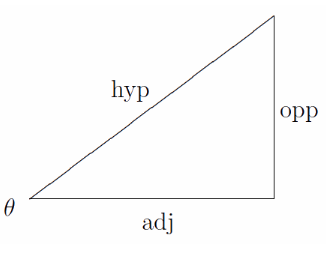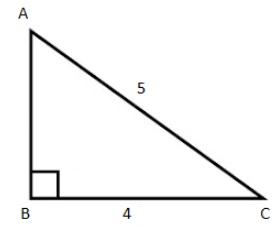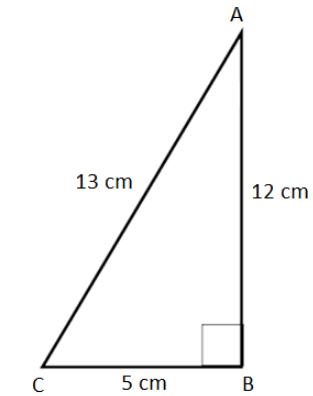# Sin Tan formula

Sin Tan Formula: The sine function and tangent function depends on the sides of the right-angled triangle. If theta is an angle in a right triangle, then;

Tan Theta = Opposite side to theta / Adjacent side to theta

sin Theta = Opposite side to theta / Hypotenuse of triangle

## Sin – Tan x formula

The Tan Theta is the ratio of the Opposite side to the Adjacent, where (Θ) is one of the acute angles.

 Tan Θ = Opposite Side/ Adjacent Side sin Θ = Opposite Side/ Hypotenuse

See the figure of a right triangle below, where theta is an angle and adjacent side (also called base), opposite side (also called perpendicular) and hypotenuse are the three sides.## Tan Sin Formula Questions

Let’s have a look at the practice question of tan theta formula.

Question 1: If Sec x = 7/4, find tan x and sin x?

Solution: As Sec x = 1/cos x

7/4 = 1/ cos x

Cos x = 4/7

Tan2x = sec2x – 1

= (7/4)2 – 1

= 49/16 – 1

= 33/16

Tan x = √(33/16)

Sin2x = 1-cos2x
= 1 – (4/7)2
= 1 – 16/49
= 33/49
Sin x = √(33/49)

Question 2: From the following figure find the value of tan A.Solution:
From the given figure,
sin A = Opposite/Hypotenuse
= BC/AC
= 4/5
By Pythagoras theorem,
AC2 = AB2 + BC2
52 = AB2 + 42
AB2 = 25 – 16
AB2 = 9
AB = √9
AB = 3
tan A = Opposite/Adjacent
= BC/AB
= 4/3

Question 3: From the given right triangle ABC, find the values of sin A, tan A, sin C, and tan C.Solution:
From the given figure,
AB = 12 cm, BC = 5 cm, AC = 13 cm
We know that,
sin x = Side opposite to angle x/Hypotenuse
tan x = Side opposite to angle x/Side adjacent to angle x
Therefore,

sin A = BC/AC = 5/13
tan A = BC/AB = 5/12
sin C = AB/AC = 12/13
tan C = AB/BC = 12/5

To practice more Trigonometric questions and understand the trigonometric concepts better, register on BYJU’S.

#### 1 Comment

1. Samin Haq

Great work! Please put more formulas of math! Thank you BYJU’s!